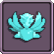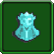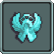## FANDOM

113 Pages

Faction Unions are upgrades available to Vanilla and Neutral factions after Ascension 2. To unlock these upgrades, you will need a faction's Leges Proof and unlock its Leges Unique Building.

• Unions Cost: 1 Qi (1E18) of that factions coins
• Upgrades Cost: 1 Nod (1E60) Emerald Coins

## Union UpgradesEdit

Name EffectBubble Swarm Increase maximum mana based on the amount of Swarming Towers you own.

Formula: $sumofdigits\ x * 100 * x^{0.45}$  where x is the number of Swarming Towers you own.Wooden Dice Increase Elven Luck chance to activate and its effects based on the amount of Arboreal Cities you own.

Elven Luck Formula: $x^{0.3}$
Production Formula: $(6 * x^{0.6})$
Faction Coin Formula: $(x^{0.7})$
where x is the number of Arboreal Cities you own.Angelic Fortitude Increase the bonus multiplier for all spells at tier 7 and above based on the amount of Holy Sites you own. All Dragon's Breath effects are increased based on the amount of Holy Sites you own.

Formula: $(0.6 * x^{0.6})$  where x is the number of Holy Sites you own.Slave Market Increase production bonus from Gem based on the amount of Slave Markets you own.

Formula: $x^{0.5}$  where x is the number of Slave Markets you own.Flesh Servants Increase assistants based on the amount of Flesh Workshops you own.

Formula: $1.2 * x^{0.65}$  where x is the number of Flesh Workshops you own.Abyssal Furnace Increase the production of all buildings based on the amount of Burning Abysses you own. Higher tiers get better bonuses.

Formula: $t^2 * x^{0.5}$ where x is Hall of legends count, t is building tier.Giant Market Increase Royal Exchanges count based on the amount of Mountain Palace you own.

Formula: $1.5x^{0.5}$  where x is the number of Mountain Palaces you own.Building Vines Increase Non-Unique building count based on the amount of Ziggurats you own.

Formula: $1.5x^{0.5}$  where x is the number of Ziggurats you own.Primal Knowledge Multiplicatively increase Faction Coin find chance based on the amount of Forbidden Libraries you own. Grows over time and resets every 15 minutes.

Formula: $(t % 900) / 60 * x^{0.4}$ where x is Forbidden Libraries count, t is time this game.

Community content is available under CC-BY-SA unless otherwise noted.